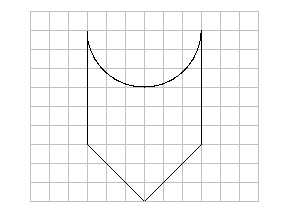Name:    Quiz 6.1-6.2

Multiple Choice
Identify the choice that best completes the statement or answers the question.

1.

A circular sinkhole has a radius of 18 meters. A week later, it has a diameter of 72 meters. How much greater is the circumference of the sinkhole compared to the previous week?
 a. 169.56 m c. 124.14 m b. 395.64 m d. 113.04 m

Find the perimeter.

2.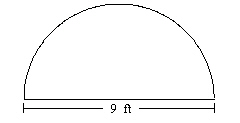3.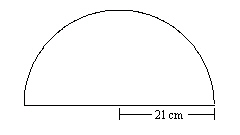Find the perimeter.

4.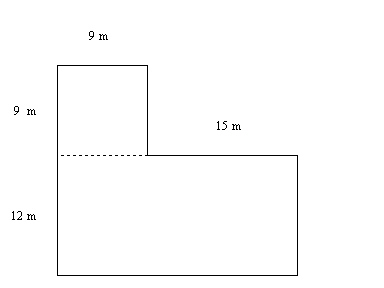a. 369 m c. 90 m b. 99 m d. 45 m

5.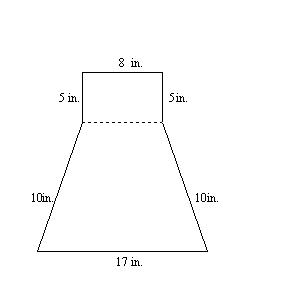a. 40 in. c. 77 in. b. 63 in. d. 55 in.

6.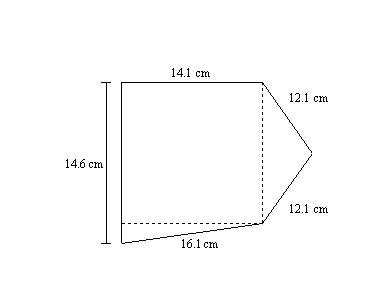a. 60.9 cm c. 83.1 cm b. 69 cm d. 70.11 cm

7.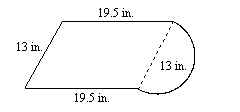a. 113.23 in. c. 78 in. b. 72.41 in. d. 73.51 in.

8.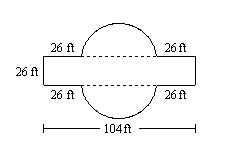a. 320.28 ft c. 293.3 ft b. 319.28 ft d. 234 ft

Numeric Response

Find the diameter.

1.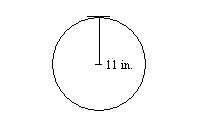Find the circumference. Use 3.14 or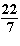for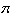.

2.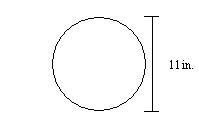3.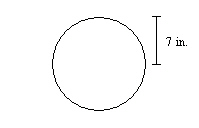Find the circumferences of both circles.

1.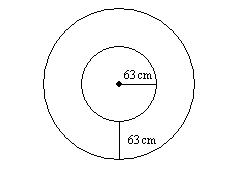Each square on the grid paper is 1 square inch.  Estimate the perimeter of the figure.

2.